GeeksforGeeks App
Open AppBrowser
Continue

# Memory representation of Binomial Heap

Prerequisites: Binomial Heap

Binomial trees are multi-way trees typically stored in the left-child, right-sibling representation, and each node stores its degree. Binomial heaps are collection of binomial trees stored in ascending order of size. The root list in the heap is a linked list of roots of the Binomial heap. The degree of the nodes of the roots increase as on traversing the root list.

The number of binomial trees in a binomial heap can be found with the binary value of the number of nodes in the binomial heap. This article focuses on memory representation of binomial heaps.

### Binomial Heap Node:

• Fields in each node:

Each node in a binomial heap has 5 fields :

1. Pointer to parent
2. Key
3. Degree
4. Pointer to child (leftmost child)
5. Pointer to sibling which is immediately to its right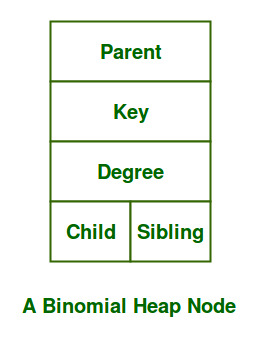• Pointers in each node:

Each node has the following pointers:

1. A parent pointer pointing to the immediate parent of the node
2. A left pointer pointing to the first child of the node
3. A right pointer pointing to the next sibling of the node.

### Types of nodes and their representations:

• Single node in the Heap: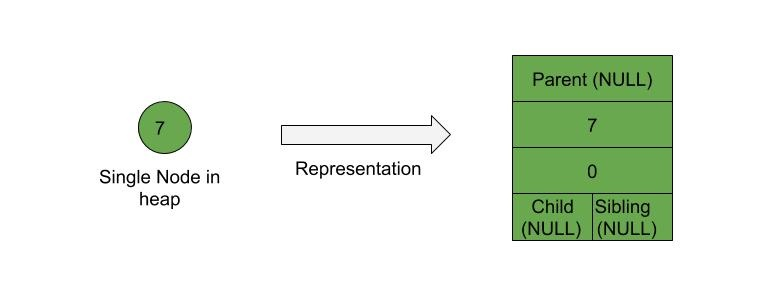• Parent – Child relationship between nodes: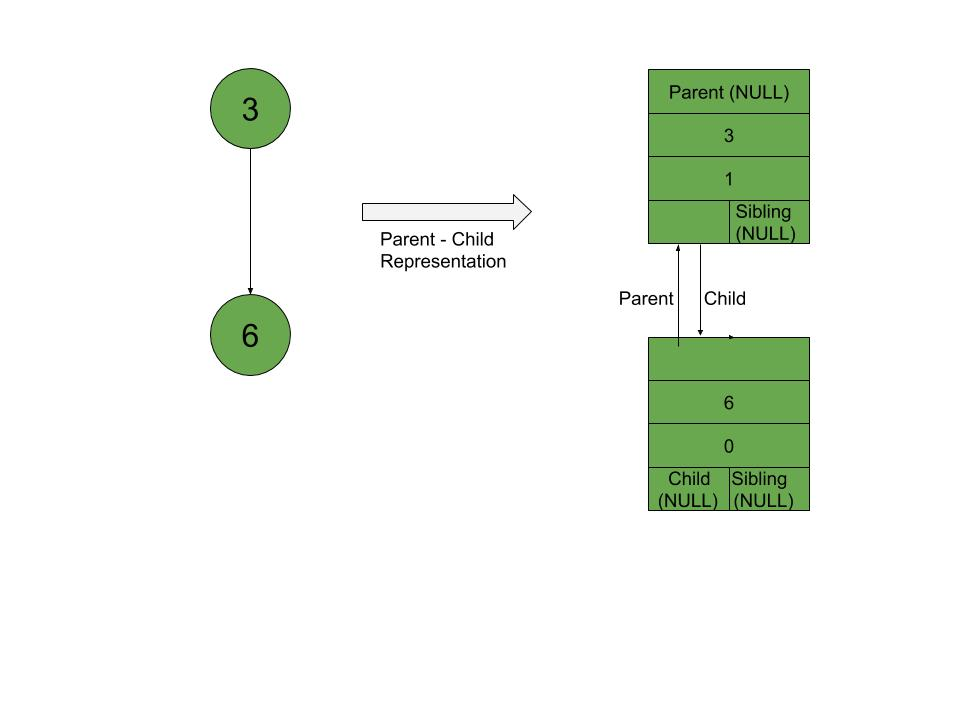• Sibling relationship between nodes: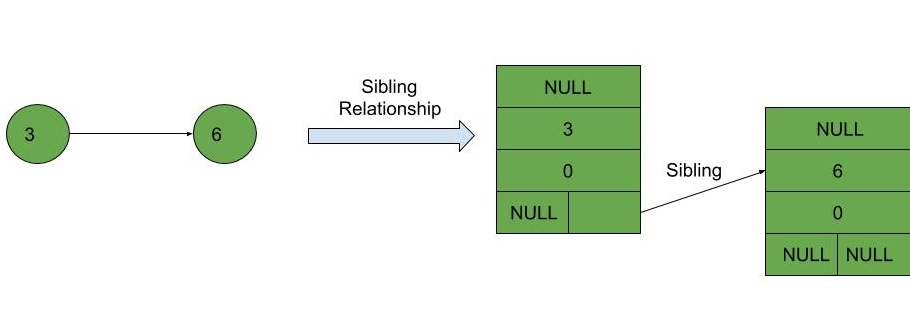### Representation of Full binomial heap: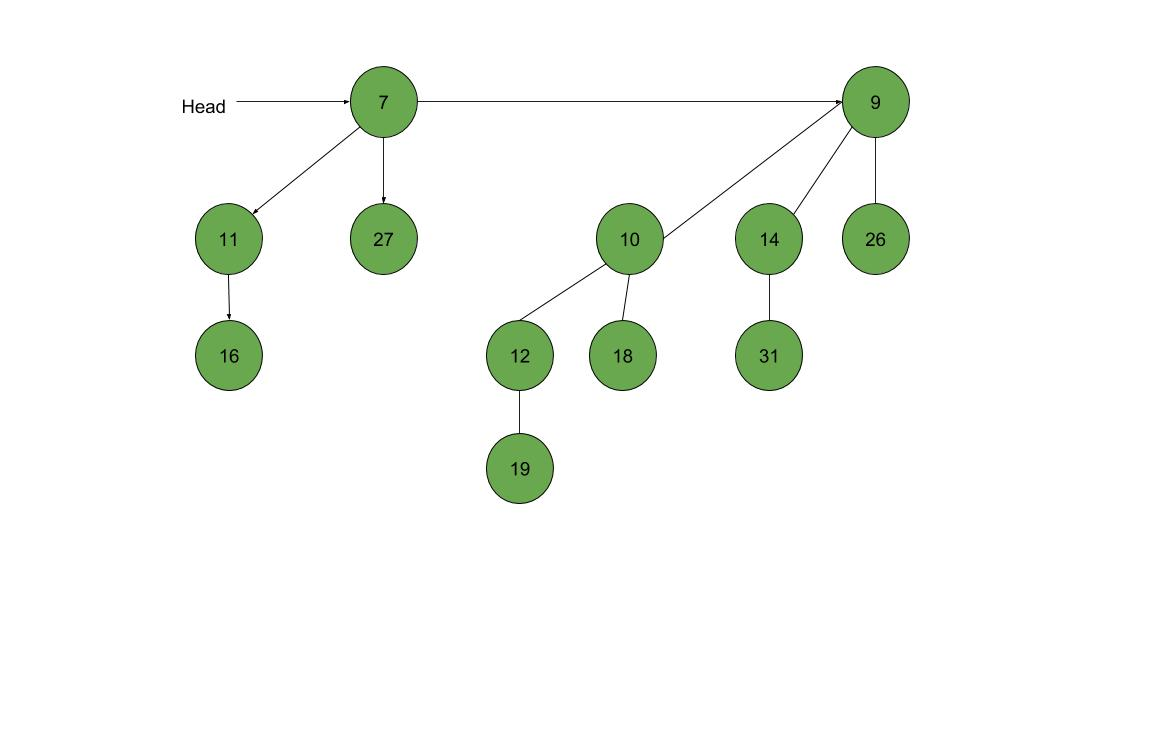The memory representation of each node of the Binomial heap given above can be illustrated using the following diagram: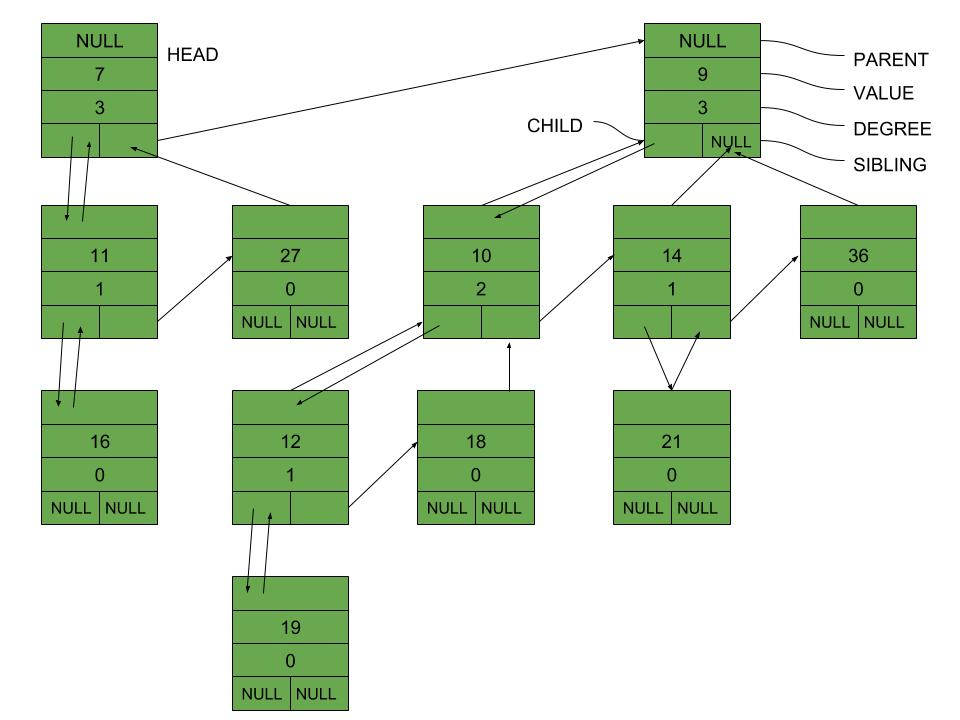My Personal Notes arrow_drop_up